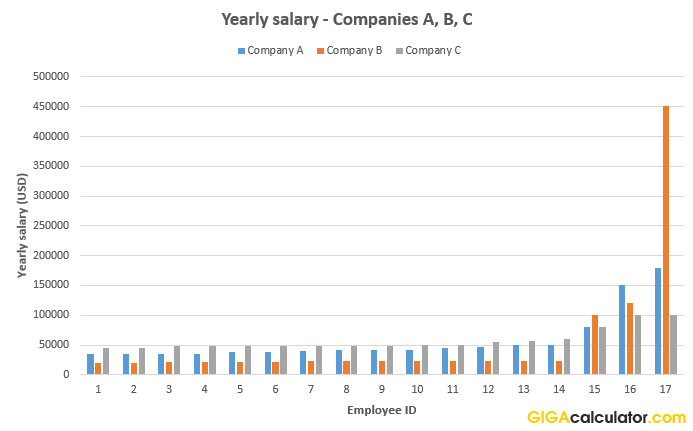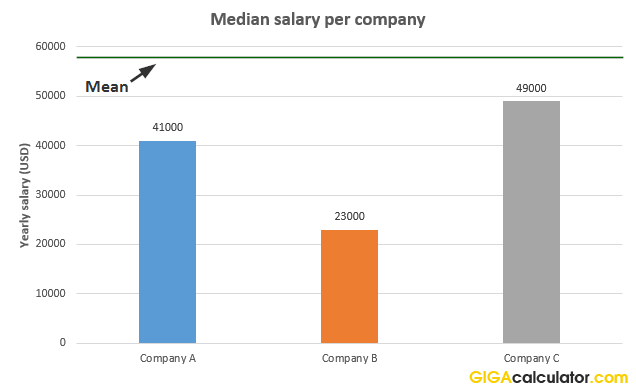# Median Calculator

Use this median calculator to easily calculate the median of a set of numbers, a.k.a. the "middle number". Median finder with detailed explanations.

Share calculator:

Embed this tool:
get code

## What is a median?

The median is a simple descriptive statistic, representing the middle value in an ordered set of numbers, separating the greater and lesser halves of the set. For a continuous probability distribution, the median value is such that a number is equally likely to be above or below it.

Finding the median is useful when one needs a value which splits a set of numbers in two halfs, respectively below and above the 50 percent mark. As a summary descriptive statistic of a given set, it has the property of demarking the 50-th percentile of its distribution.

## How to calculate the median?

To find the median of a set of numbers, first, order them from the lowest to the highest (highest to lowest works just as well). Second, determine of the elements of the set are an odd or even number. Third, if the number of data points is odd, there is always a middle point, e.g. in the set 1, 2, 3, 4, 5 it is 3. In sets of even number of elements, the median is the arithmetic mean of the two points next to the midpoint, for example in the set 1, 2, 3, 4 the median is between 2 and 3, so it is (2 + 3) / 2 = 2.5. Using our median calculator, of course, it takes care of all three steps for you.

## Useful properties and practical applications

One reason to prefer the median is that it is skewed less by extreme values (large or small) and in some cases is a better approximation to a "typical" value. For this reason, it is mainly used in skewed distributions where it summarizes the data set differently than both the mean and the mode. When thinking in percentiles, the median is the 2nd quartile, 5th decile and 50th percentile. However, the choice of what average to use should be informed primarily by the question asked of the data as explained in detail in When to Use Mean, Median, or Mode.

Statistical agencies often report median household income and base some indices on medians. For example, the US Center for Disease Control (CDC) reports median height and weight values for boys and girls between the ages 0-21 while the arithmetic mean is more often used for adults.

Let us see an example where the median is more informative than the mean. These are the salaries of 3 companies with 17 employees each:Despite the obvious differences, all three have the same mean: \$57,705.88, which an outsider without access to the full data and poor understanding of statistics might interpret to mean they are essentially the same. The medians, however, calculated using our median calculator, reveal a much different picture:The medians show us that in Company B 50% of salaries are above just \$23,000, while in company A half of the salaries are above \$41,000 and in Company C is even better with half of workers getting \$49,000 or more. So, if you had the choice to become an employee of one of these companies and would know that your salary would be randomly assigned based off existing salaries, then based on the median your best bet from the point of minimizing the risk for a very low salary, is company C. However, if you want to take the downside risk and enjoy the upside gains, you might choose company B, as there you have a chance to end up with a \$450,000 yearly pay. You would have no such advantage if you were only looking at the arithmetic mean.

Of course, the above example is just an illustration - there are many cases where the median is no better or worse than the mean, or they might coincide, so the choice of statistic should be as informed as possible.

#### Cite this calculator & page

If you'd like to cite this online calculator resource and information as provided on the page, you can use the following citation:
Georgiev G.Z., "Median Calculator", [online] Available at: https://www.gigacalculator.com/calculators/median-calculator.php URL [Accessed Date: 27 Mar, 2023].